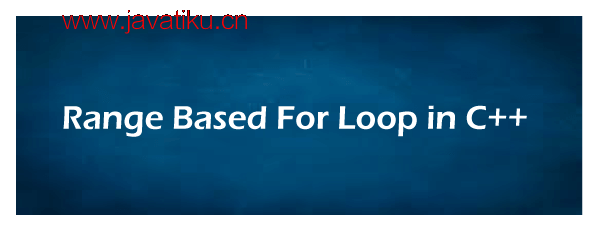# C++教程-C++中的范围-based for循环C++中的范围-based for循环``for (range_declaration : range_expression) 循环语句 ``
• range_declaration：用于声明一个变量，其类型与range_expression表示的集合元素的类型相同或引用该类型。
• range_expression：定义表示适当序列的表达式。
• 循环语句：定义范围-based for循环的主体，其中包含一个或多个语句，这些语句将重复执行，直到range_expression结束。

### 使用范围-based for循环打印数组的每个元素的程序

program.cpp

``````#include <iostream>
using namespace std;
int main ()
{
int arr1  = { 10, 20, 30, 40, 50};
double darr  = { 2.4, 4.5, 1.5, 3.5, 4.0 };

// 使用范围-based for循环
for (const auto &var : arr1)
{
cout << var << " ";
}
// 使用auto关键字自动指定darr容器的数据类型。
for (const auto &var : darr)
{
cout << var << " ";
}
return 0;
}``````

``````10 20 30 40 50
2.4 4.5 1.5 3.5 4.0``````

### 使用范围-based for循环演示向量

Program2.cpp

``````#include <iostream>
#include <vector>
using namespace std;

int main()
{
int x; // 声明整数变量
// 声明向量变量
vector<int> vect = {5, 10 , 25, 20, 25};

// 显示向量元素
for (int x : vect)
{
cout << x << " ";
}
return 0;
}``````

``5 10 25 20 25``

### 使用范围-based for循环打印数组的程序（带有引用）

Program3.cpp

``````#include <iostream>
#include <array>
#include <cstdlib>
using namespace std;

int main(){
array<int, 7> data = {1, 3, -2, 4, 6, 7, 9};
cout << " 在更新元素之前：" << endl;
for (int x : data){
cout << x << " ";
}
// 传递引用
for (int &itemRef : data){
itemRef *= 3;
}
cout << endl << " 更新元素之后：" << endl;
for (int x : data){
cout << x << " ";
}
cout << endl;
return 0;
}``````

``````在更新元素之前：
1 3 -2 4 6 7 9

3 9 -6 12 18 21 27``````

### 嵌套的范围-based for循环

``````for (int x : range_expression) // 外部循环
{
for (int y : range_expression) // 内部循环
{
// 要执行的语句
}
// 要执行的语句
} ``````

### 在C++中打印嵌套的范围-based for循环的程序

Range.cpp

``````#include <iostream>
using namespace std;
int main ()
{
int arr1  = { 0, 1, 2, 3 };
int arr2 = { 1, 2, 3``````

Range.cpp

``````#include <iostream>
using namespace std;
int main ()
{
int arr1 = { 0, 1, 2, 3 };
int arr2 = { 1, 2, 3, 4, 5 };
// 使用嵌套的范围循环 for (int x : arr1)
{
// 声明嵌套循环 for (int y : arr2)
{
cout << " x = " << x << " and j = " << y << endl;
}
}
return 0;
}``````

``````x = 0 and j = 1
x = 0 and j = 2
x = 0 and j = 3
x = 0 and j = 4
x = 0 and j = 5
x = 1 and j = 1
x = 1 and j = 2
x = 1 and j = 3
x = 1 and j = 4
x = 1 and j = 5
x = 2 and j = 1
x = 2 and j = 2
x = 2 and j = 3
x= 2 and j = 4
x = 2 and j = 5
x = 3 and j = 1
x = 3 and j = 2
x = 3 and j = 3
x = 3 and j = 4
x = 3 and j = 5``````

### 传统的for循环和基于范围的for循环有什么区别？

``````for (变量初始化; 指定条件; 更新计数器)
{
// 要执行的语句；
} ``````

``````for (范围声明 : 范围表达式)
{
循环语句；
// 要执行的语句；
} ``````

### 基于范围的for循环的优点

• 易于使用，语法简单。
• 基于范围的for循环不需要计算容器中元素的数量。
• 它能识别容器的起始和结束元素。
• 我们可以轻松修改容器的大小和元素。
• 它不会创建元素的副本。
• 它比传统的for循环更快。
• 它通常使用auto关键字来识别容器元素的数据类型。

### 基于范围的for循环的缺点

• 无法遍历列表的部分元素。
• 无法以逆序遍历。
• 无法在指针上使用。
• 无法获取当前元素的索引值。